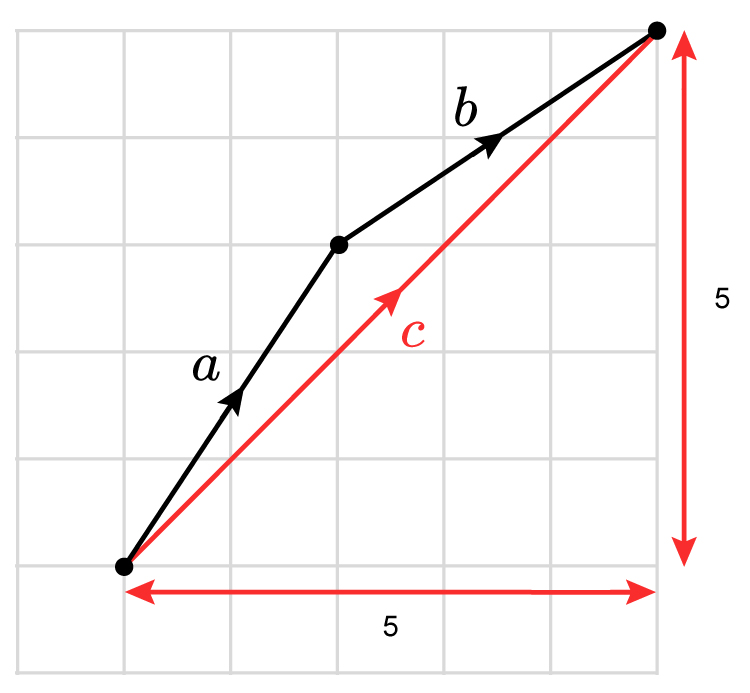Add just like any normal calculation but keep the top row separate from the bottom row

Example 1

Add vector a  to vector b

vector a=((2),(3))  vector b=((3),(2))

vector a+b=((2+3),(3+2))=((5),(5))

Shown graphically this isvector c=((5),(5))